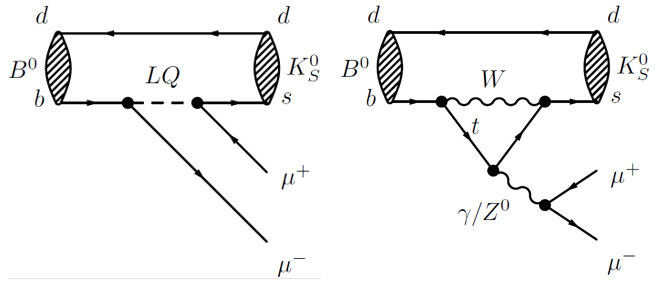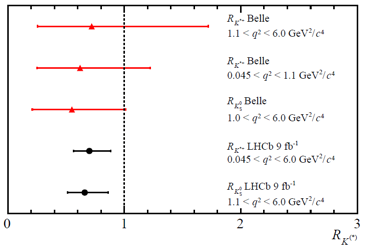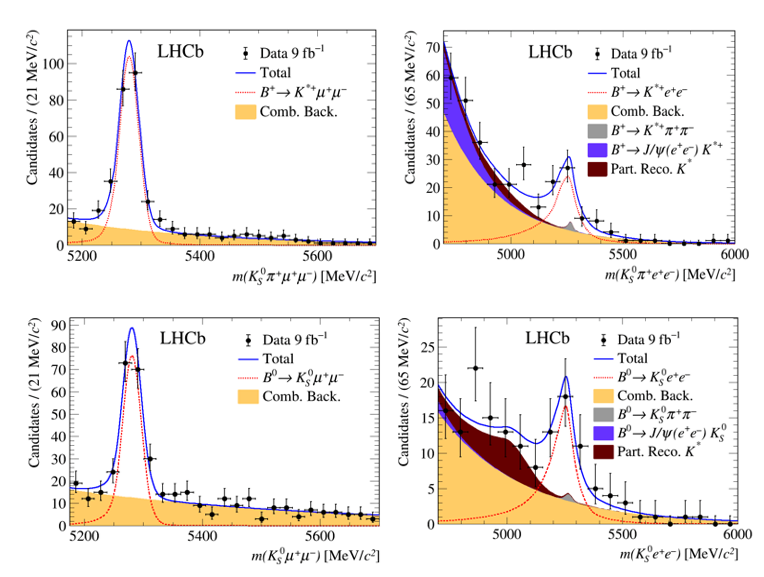Date

Test of lepton universality using B0KS0l+l- and B+K*+l+l- decays.

###Today at the CERN seminar and at the Rencontres de Blois the LHCb Collaboration presented new tests of lepton universality, one of the basic principles of the Standard Model (SM) of particle physics. This principle states that the SM treats the three charged leptons (electrons, muons and taus) identically, except for differences due to their different masses. The new measurements, while of limited statistical sensitivity, exhibit the same coherent pattern of deviations from lepton universality as seen in previous LHCb results.

In March 2021, the collaboration reported an evidence at 3.1 standard deviations (σ) for the breaking of lepton universality in a measurement of the ratio RK. The ratio RK describes how often a B+ meson decays to a charged kaon and either a positive and a negatively charge muon (K+μ+μ-) or a positron-electron pair (K+e+e-). These decays are extremely rare, occurring at a rate of only one in two million B+ meson decays. The decays involve the transformation of a beauty quark into a strange quark (b→s), a process that is highly suppressed in the Standard Model and can be affected by the existence of new particles, which could have masses too high to be produced directly at the Large Hadron Collider. The left graph shows Standard Model contributions involving γ, W+, and Z0 bosons. The right one shows a possible new physics contribution to the decay with a hypothetical leptoquark (LQ) which, unlike the Standard Model bosons, could have different interaction strengths with the different types of leptons.

Deviations at the level of 2.2-2.5 σ in a measurement of the ratio RK*0 were also reported in April 2017.

Both ratios exhibit a deficit of μ+μ- pairs with respect to e+e- pairs. Todays results also report tests of lepton universality, using ratios RK and RK*. However, in this measurement, K and K* mesons with different electric charges have been used. Physicists call these newly measured decays theisospin partners” of the previously measured decays. The new ratios are labelled RKS0 and RK*+.

LHCb has studied a number of other such ratios comparing decays with different leptons (l=e, μ, or τ leptons) in beauty particle decays (see R(D*)R(J/ψ) and R(pK)). These results revealed hints of deviations from lepton universality, none of which is significant enough to constitute an observation of new physics on its own. However, according to theorists who study possible extensions of the SM, these deviations combined suggest an interesting and coherent pattern, which could be evidence for physics beyond the SM.

The values of the RKS0 and RK*+ ratios are presented at the top of this article. These ratios correspond to the transitions B0→KS0l+l- and B+→K*+l+l-.Figure 1. Quark diagrams for a B0→KS0l+l- decay.

The ratios are also presented in figure 2 for different regions of the dilepton invariant mass squared, q2. They are individually consistent with the SM at the 1.4 and 1.5σ level, their combination at the 2σ level (for experts: using a fit for a single Wilson coefficient). It is interesting to note that the central values exhibit the same coherent pattern of deviation as the other lepton universality tests performed by LHCb – the muon pairs are observed less frequently than the electron pairs. The image to the left lists the new measurements by LHCb, the most precise to date, of RKS0 and RK*+ ratios, and previous measurments by Belle.Figure 2. RKS0 and RK*+ Ratios for different regions of the dilepton invariant mass squared, q2

The bottom left image above shows the KS0μ+μ- mass distribution and the bottom right image the KS0e+e- mass used to determine the RKS0 ratio. The top left image shows the KS0π+ μ+μ- mass distribution and the top right the KS0π+e+e- mass distribution used to determine the RK*+ ratio. Note that a K*+ meson decays into a KS0 and a π+ meson and note also a clear accumulation of events at the B0 or B+ mass.The Institute of Cosmos Sciences of the University of Barcelona participates in this experiment with the researchers Carla Marín, Eugeni Graugés, Lluís Garrido, Ricardo Vázquez who have contributed to the development and optimisation of the electron reconstruction process, which is a crucial ingredient in the obtention of these measurements. We should also mention that the ICC-LHCb group counts with a new addition to their team, PhD Student Albert López, who will work on a similar Lepton Universality measurements as described in this news item with baryons. He will analyse the transition Λb0→pK-l+l-

Read more in the LHCb presentations at CERN and at Blois, and in the LHCb paper. You can also stay on the loop of the announcements of new results by following the experiment’s twitter account @LHCbExperiment.